# CDS 2015 Maths Question Paper -2

1. The number of values of x satisfying x + 100/x > 50, where x is a natural number less than or equal to 100 is
• 51
• 53
• 55
• 57
1. What is the sum of digits of the least multiple of 13, which when divided by 6, 8 and 12 leaves 5, 7 and 11 respectively as the remainders ?
• 5
• 6
• 7
• 8
1. A milkman claims to sell milk at its cost price only. Still he is making a profit of 20% since he has mixed some amount of water in the milk. What is the percentage of milk in the mixture ?
• 200/3%
• 75%
• 80%
• 250/3%
1. What is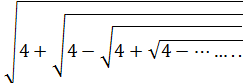equal to ?
• 3
• √13-1/2
• √13+1/2
• 0
1. The largest natural number which divides every natural number of the form (n3 – n)(n – 2), where n is a natural number greater than 2 is
• 6
• 12
• 24
• 48
1. The average of m numbers is n4 and the average of n numbers is m4. The average of (m + n) numbers is
• mn
• m2+ 2
• mn(m2+n2)
• mn(m2 + n2 – mn)
1. The digit in the units place of the resulting number of the expression (234)100 + (234)101 is
• 6
• 4
• 2
• 0
1. If = √3 + √2, then the value of x3+x+1/x+1/x3
• 10√3
• 20√3
• 10√2
• 20√2
1. The value of 1/1×4+1/ 4×7 +1/7×10+…….+1/16×19 is.
• 5/19
• 6/19
• 8/19
• 9/19
1. Two trains are moving in the same direction at 1.5 km/minute and 60 km/ hour respectively. A man in the faster train observes that it takes 27 seconds to cross the slower train. The length of the slower train is
• 225 m
• 230 m
• 240 m
• 250 m
1. A tin of oil was 4/5 full. When 6 bottles of oil were taken out from this tin and 4 bottles of oil were poured into it, it was ¾ full. Oil of how many bottles can the tin contain ? (All bottles are of equal volume)
• 35
• 40
• 45
• 50
1. A number when divided by 7 leaves a remainder 3 and the resulting quotient when divided by 11 leaves a remainder 6. If the same number when divided by 11 leaves a remainder m and the resulting quotient when divided by 7 leaves a remainder n. What are the values of m and n respectively ?
• 1 and 4
• 4 and 1
• 3 and 6
• 6 and 3
1. Consider the following in respect of the equation y =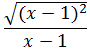2. y = 1 if x > 1
3. y = -1 if x < 1
4. y exists for all values of x

Which of the above statements is/are correct ?

• 1 only
• 2 only
• 1 and 2 only
• 1, 2 and 3
1. If (x + 1) is the HCF of Ax1 + Bx + C and Bx2 + Ax + C where A ≠ B, then the value of C is
• A
• B
• A-B
• 0
1. The seven digit number 876p37q is divisible by 225. The values of p and q can be respectively
• 9,0
• 0, 0
• 0, 5
• 9,5
1. If a, b and c satisfy the equation x3 – 3x2 + 2x + 1 = 0 then what is the value of 1/a+1/b+1/c ?
• -1/2
• 2
• -2
• ½
1. A and B are two taps which can fill a tank individually in 10 minutes and 20 minutes respectively. However, there is a leakage at the bottom, which can empty a filled tank in 40 minutes. If the tank is empty initially, how much time will both the taps take to fill the tank with leakage ?
• 2 minutes
• 4 minutes
• 5 minutes
• 8 minutes
1. Which one of the following is correct ?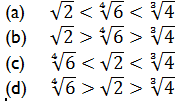1. The value of a single discount on some amount which is equivalent to a series of discounts of 10%, 20% and 40% on the same amount, is equal to
• 2%
• 50%
• 8%
• 70%
1. If k = x-y + 2z where -2 ≤ x ≤ 1,-1 ≤ y ≤ 2 and 3 ≤ z ≤ 6, then which one of the following is correct ?
• 0 ≤ k ≤ 9
• 5 ≤ k ≤ 11
• 2 ≤ k ≤ 14
• 2 ≤ k ≤ 11
1. The number of pairs (x, y) where x, y are integers satisfying the equation 21x + 48y = 5 is
• Zero
• One
• Two
• Infinity
1. Let x and y be positive integers such that x is prime and y is composite.

Which of the following statements are correct ?

1. (y-x) can be ah even integer.
2. xy can be an even integer.
3. 5(x+y) can be an even integer.

Select the correct answer using the code given below:

• 1 and 2 only
• 2 and 3 only
• 1 and 3 only
• 1,2 and 3
1. Let x and y be positive integers such that x > y. The expressions 3x + 2y and 2x + 3y when divided by 5 leave remainders 2 and 3 respectively. What is the remainder when (x-y) is divided by 5?
• 4
• 2
• 1
• 0
1. In a race A, B and C take part. A beats B by 30 m, B beats C by 20 m and A beats C by 48 m. Which of the following is/are correct ?
2. The length of the race is 300 m.
3. The speeds of A, B and C are in the ratio 50 : 45 : 42.

Select the correct answer using the code given below :

• 1 only
• 2 only
• Both 1 and 2
• Neither 1 nor 2
1. If the roots of the quadratic equation x2-4x –log10 N = 0 are all real, then the minimum value of N is
• 1/100
• 1/1000
• 1/10000
• 10000
1. A motor boat, whose speed is 15 km/ hour in still water goes 30 km down- stream and comes back in a total of 4 hour and 30 minutes. The speed of the stream is
• 4 km/hour
• 5 km/hour
• 6 km/hour
• 10 km/hour
1. If 4 men working 4 hours per day for 4 days complete 4 units of work, then how many units of work will be completed by 2 men working for 2 hours per day in 2 days ?
• 2
• 1
• ½
• 1/8
1. By increasing the speed of his car by 15 km/hour, a person covers 300 km distance by taking an hour less than before. The original speed of the car was
• 45 km/hour
• 50 km/hour
• 60 km/hour
• 75 km/hour
1. If m persons can paint a house in d days, how many days will it take for (m + 2) persons to paint the same house ?
• md + 2
• md-2
• m+2/md
• md/m+2
1. If a: b = 3:5 and b : c = 7:8, then 2a :3b : 1c is equal to
• 42 : 105 : 320
• 15 : 21 : 35
• 6 : 15 : 40
• 30 : 21 : 350′
1. Two trains, one is of 121 m in length at the speed of 40 km/hour and the other is of 99 m in length at the speed of 32 km/hour are running in opposite directions. In how much time will they be completely clear from each other from the moment they meet ?
• 10 s
• 11s
• 16 s
• 21 s
1. Three athletes run a 4 km race. Their speeds are in the ratio 16 : 15 : 11. When the winner wins the race, then the distance between the athlete in the second position to the athlete in the third position is
• 1000 m
• 800 m
• 750 m
• 600 m
1. The sum of first 47 terms of the series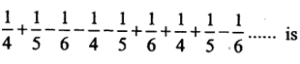• 0
• -1/6
• 1/6
• 9/20
1. Which one of the following is correct ?
• (x + 2) is a factor of x4-6x3 +12x2-24x + 32
• (x + 2) is a factor of x4 + 6x3 – 12x2 + 24x – 32
• (x-2) is a factor of x4 – 6x3 + 12x2 – 24x+ 32
• (x-2) is a factor of x4 + 6x3– 12x2 + 24x-32
1. 20% of a number when added to 20 becomes the number itself, then the number is
• 20
• 25
• 50
• 80
1. Consider the following statements :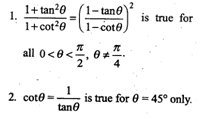Which of the above statements is/are correct ?

• 1 only
• 2 only
• Both 1 and 2
• Neither 1 nor 2
1. If x = a cosθ and y = b cotθ , then (ax-1 – by -1+ by-l) is equal to
• 0
• 1
• tan2 θ
• sin2 θ
1. cosθ /1-sinθ  is equal to (where θ ≠π/2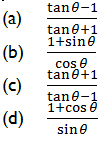1. If tan(x + 40)°tan(x + 20)°tan(3x)°tan(70 -x)°tan(50 -x)° = 1, then the value of x is equal to
• 30
• 20
• 15
• 10
1. If θ is an acute angle and sinθ  cosθ  =2cos3 θ – l.5cosθ , then what is sin θ  equal to ?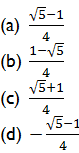1. Consider the following statements :
2. sin66° is less than cos66°.
3. sin26° is less than cos26°.

Which of the above statements is/are correct ?

• 1 only
• 2 only
• Both 1 and 2
• Neither 1 nor 2
1. If a and b are positive, then the relation sinθ =2a + 3b/3b is  :
• not possible
• possible only if a – b
• possible if a > b
• possible if a < b
1. An aeroplane flying at a height of 3000 m passes vertically above another aeroplane at an instant when the angles of elevation of the two planes from some point on the ground are 60° and 45° respectively. Then the vertical distance between the two planes is
• 1000(√3-1) m
• 1000√3 m
• 1000(3-√3) m
• 3000√3 m
1. A pole is standing erect on the ground which is horizontal. The tip of the pole is tied tight with a rope of length √12 m to a point on the ground. If the rope is making 30° with the horizontal, then the height of the pole is
• 2√3 m
• 3√2 m
• 3 m
• √3 m
1. If tan θ+ sin θ = 2, then tan θ is equal to
• ¾
• 5/4
• 3/2
• 5/2
1. A’s salary was increased by 40% and then decreased by 20%. On the whole A’s salary
• 60%
• 40%
• 20%
• 12%
1. A number consists of two digits, whose sum is 7. If the digits are reversed, the number is increased by 27. The product of digits of the number is
• 6
• 8
• 10
• 12
1. In a race of 100 m, A beats B by 4 m and A beats C by 2 m. By how many metres (approximately) would C beat B in another 100 m race assuming C and B run with their respective speeds as in the earlier race ?
• 2
• 04
• 08
• 2
1. In an election 10% of the voters on the voter list did not cast their vote and 60 voters cast their ballot papers blank. There were only two candidates. The winner was supported by 47% of total voters in the voter list and he got 308 votes more than his rival. The number of voters on the voter list is
• 3600
• 6200
• 6028
• 6400
1. Consider all positive two digit numbers each of which when divided by 7 leaves a remainder 3. What is their sum ?
• 661
• 666
• 676
• 111
1. A pipe with square cross-section is supplying water to a cistern which was initially empty. The area of cross-section is 4 cm2 and the nozzle velocity of water is 40 m/s. The dimensions of the cistern are 10 m x 8 m x 6 m. Then the cistern will be full in
• 5 hours
• 9 hours
• 8 hours 20 minutes
• 8 hours
1. A hollow cylindrical drum has internal diameter of 30 cm and a height of 1 m. What is the maximum number of cylindrical boxes of diameter 10 cm and height 10 cm each that can be packed in the drum ?
• 60
• 70
• 80
• 90
1. The speeds of three buses are in the ratio 2:3:4. The time taken by these buses to travel the same distance will be in the ratio
• 2:3:4
• 4:3:2
• 4:3:6
• 6:4:3
1. A square and an equilateral triangle have equal perimeter. If the diagonal of the square is 12√2 cm, then the area of the triangle is
• 24√2 cm2
• 24√3 cm2
• 48√3 cm2
• 64√3 cm2

1. A boy is cycling such that the wheels of the cycle are making 140 revolutions per minute. If the radius of the wheel is 30 cm, the speed of the cycle is
• 5 km/hour
• 84 km/hour
• 16 km/hour
• 36 km/hour
1. There are 437 fruit plants in an orchard planted in rows. The distance between any two adjacent rows is 2 m and the distance between any two adjacent plants is 2 m. Each row has the same number of plants.- There is 1 m clearance on all sides of the orchard. What is the cost of fencing the area at the rate of Rs. 100 per metre ?
• 15,600
• 16,800
• 18,200
1. ABCD is a parallelogram with AB and AD as adjacent sides. If ∠A = 60° and AB = 2AD, then the diagonal BD will be equal to
1. The point O is equidistant from the three sides of a triangle ABC. Consider the following statements:
2. ∠OAC + ∠OCB + ∠OBA = 90°
3. ∠BOC=2 ∠BAC
4. The perpendiculars drawn from any point on OA to AB and AC are always equal

Which of the above statements are correct ?

• 1 and 2 only
• 2 and 3 only
• 1 and 3 only
• 1, 2 and 3
1. Consider the following statements :
2. If the height of a cylinder is doubled, the area of the curved surface is doubled.
3. If the radius of a hemispherical solid is doubled, its total surface area becomes fourfold.

Which of the above statements is/are correct ?

• (a) 1 only
• (b) 2 only
• (c) Both 1and 2
• (d) Neither 1 nor 2
1. A large water tank has the shape of a cube. If 128 m3 of water is pumped out, the water level goes down by 2 m. Then the maximum capacity of the tank is
• 512 m3
• 480 m3
• 324 m3
• 256 m3

1. The circumference of a circle is 100 cm. The side of the square inscribed in the circle is
• 50√2 cm
• 100/π cm
• 50√2/π cm
• 100√2/π cm
1. From the solid gold in the form of a cube of side length 1 cm, spherical solid balls each having the surface area π 1/3 cm2 are to be made. Assuming that there is no loss of the material in the process of making the balls, the maximum number of balls made will be
• 3
• 4
• 6
• 9
1. Water is filled in a container in such a manner that its volume doubles every 5 minutes. If it takes 30 minutes for the container to be full, in how much time will it be one-fourth full ?
• 5 minutes
• 15 minutes
• 20 minutes
• 5 minutes
1. 30 metallic cylinders of same size are melted and cast in the form of cones having the same radius and height as those of the cylinders.

Consider the following statements :

Statement I : A maximum of 90 cones will be obtained.

Statement II : The Curved surface of the cylinder can be flattened in the shape of a rectangle but the curved surface of  the cone when flattened has the shape of triangle,.

Which one of the following is correct in respect of the above ?

• Both Statement I and Statement II 1 are correct and Statement II is the correct explanation of Statement I
• Both Statement I and Statement II are correct and Statement II is not the correct explanation of Statement I
• Statement I is correct but Statement II is not correct
• Statement I is not correct but Statement II is correct
1. An equilateral triangle BOC is drawn inside a square ABCD. If angle AOD =2θ , what is tan θ equal to ?
• 2-√3
• 1+√2
• 4-√3
• 2 +√3
1. The diameter of a wheel that makes 452 revolutions to move 2 km and 26 decametre is equal to
• 1 9/22 m
• 1 13/22 m
• 2 5/11 m
• 2 7/11 m
1. If X is any point within a square ABCD and on AX a square AXYZ is described, which of the following is/are correct ?

BX= DZ or BZ = DX

Select the correct answer using the code given below:

• 1only
• 2 only
• Both 1 and 2
• Neither 1 nor 2
1. The two adjacent sides of a cyclic quadrilateral are 2 cm and 5 cm and the angle between them is 60°. If the third side is 3 cm, then the fourth side is of length
• 2 cm
• 3 cm
• 4 cm
• 5 cm
1. Let A denote the set of quadrilaterals having two diagonals equal and bisecting each other. Let B denote the set of quadrilaterals having diagonals bisecting each other at 90°. Then A ∩ B denotes
• the set of parallelograms
• the set of rhombuses
• the set of squares
• the set of rectangles
1. A square is inscribed in a right triangle with legs x and y and has common right angle with the triangle. The perimeter of the square is given by
• 2xy/x + y
• 4xy/x + y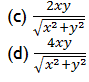1. From a rectangular sheet of sides 18 cm and 14 cm, a semicircular portion with smaller side as diameter is taken out. Then the area of the remaining sheet will be
• 98 cm2
• 100 cm2
• 108 cm2
• 175 cm2

1. ABCD is a square. If the sides AB and CD are increased by 30%, sides BC and AD are increased by 20%, then the area of the resulting rectangle exceeds the area of the square by
• 50%
• 52%
• 54%
• 56%
1. A water tank, open at the top, is hemi- spherical at the bottom and cylindrical above it. The radius is 12 m and the capacity is 3312n m3. The ratio of the surface areas of the spherical and cylindrical portions is
• 3:5
• 4:5
• 1:1
• 6:5
1. The areas of three mutually perpendicular faces of a cuboid are x, y, z. If V is the volume, then xyz is equal to
• V
• V2
• 2V
• 2 V2
1. Let V be the volume of ah inverted cone with vertex at origin and the axis of the cone is along positive y-axis. The cone is filled with water up to half of its height. The volume of water is
• V/8
• V/6
• V/3
• V/2
1. Three rectangles R1, R2 and R3 have the same area. Their lengths x1,x2 and x3 respectively are such that x1, < x2 < x3. If V1, V2 and V3 are the volumes of the cylinders formed from the rectangles R1,R2 and R3 respectively by joining the parallel sides along the breadth, then which one of the following is correct ?
• V3< V2 < V1
• V1 < V3 < V2
• V1< V2 < V3
• V3 < V1 < V2
2. If the surface area of a cube is 13254 cm2, then the length of its diagonal is
• 44√2 cm
• 44√23 cm
• 47√2 cm
• 47 cm
1. The area of a trapezium is 336 cm2. If its parallel sides are in the ratio 5 : 7 and the perpendicular distance between them is 14 cm, then the smaller of the parallel sides is
• 20 cm
• 22 cm
• 24 cm
• 26 cm
1. How many spherical bullets each of 4 cm in diameter can be made out of a cube of lead whose edge is 44 cm ?
• 2541
• 2551
• 2561
• 2571
1. A river 2.5 m deep and 45 m wide is flowing at the speed of 3.6 km/hour. The amount of water that runs into the sea per minute is
• 6650 m3
• 6750 m3
• 6850 m3
• 6950 m3

1. AB is a line segment of length 2a, with M as mid-point. Semicircles are drawn on one side with AM, MB and AB as diameter as shown in the above figure. A circle. with centre O and radius r is drawn such that this circle touches all the three semicircles. The value of r is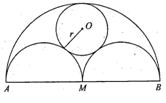• 2a/3
• a/2
• a/3
• a/4
1. From an external point P tangents PA and PB are drawn to the circle as shown in the above figure. CD is the tangent to the circle at E. If AP = 16 cm, then the perimeter of the triangle PCD is equal to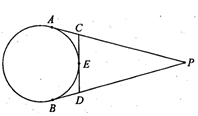• 24 cm
• 28 cm
• 30 cm
• 32 cm
1. In the above figure, ABCD is a parallelogram. P is a point on BC such that PB : PC =1:2. DP and AB when both produced meet at Q. If area of triangle BPQ is 20 square unit, the area of triangle DCP is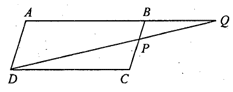• 20 square unit
• 30 square unit
• 40 square unit
• None of the above
1. Chord CD intersects the diameter AB of a circle at right angle at a point P in the ratio 1 : 2. If diameter of circle is d, then CD is equal to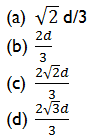1. A circle of radius r is inscribed in a regular polygon with n sides (the circle touches all sides of the polygon). If the perimeter of the polygon is p, then the area of the polygon is
• (p + n)r
• (2p-n)r
• Pr/2
• None of these
1. The LCM of two numbers is 12 times their HCF. The sum of HCF and LCM is 403. If one of the numbers is 93, then the other number is
• 124
• 128
• 134
• 138
1. The value of (0.63 + 0. 37) is
• 1
• 100/91
• 100/99
• 1000/999
1. A sum of Rs. 10,000 is deposited for 1 year at the rate of interest 10% com- pounded half yearly. What will be the interest at the end of one year ?
• 1000
• 1025
• 1050
• 1100
1. In a mixture of milk and water of volume 30 litre, the ratio of milk and water is 7:3. The quantity of water to be added to the mixture to make the ratio of milk and water 1:2 is
• 30
• 32
• 33
• 35
1. The difference of maximum values of the expressions (6 + 5x – x2) and (y – 6-y2) for any real values of x and y is
• 16
• 17
• 18
• 19
1. A clock is started at noon. By 10 minutes past 5, through what angle, the hour hand moves ?
• 160°
• 145°
• 150°
• 155°
1. The minimum value of cos2x + cos2y – cos2z is
• -1
• 0
• 1
• 2
1. The value of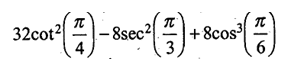is equal to.
• √3
• 2√3
• 3
• 3√3
1. If x = 91/216, then the value of 3-1/(1-x)1/3 is
• 9/5
• 5/9
• 4/9
• 4/5
1. How many right angled triangles can be formed by joining the vertices of a cuboid ?
• 24
• 28
• 32
• None of the above
1. The average weight of students in a class is 43 kg. Four new students are admitted to the class whose weights are 42 kg, 36.5 kg, 39 kg and 42.5 kg respectively. Now the average weight of the students of the class is 42-5 kg. The number of students in the beginning was
• 10
• 15
• 20
• 25
1. Four years ago, the average age of A and B was 18 years. Now the average age of A, B and C is 24 years. After 8 years, the age of C will be
• 32 years
• 28 years
• 36 years
• 40 years
1. If a variable takes discrete values a + 4, a-3.5, a -2.5, a- 3, a-2, a + 0.5, a + 5 and a – 0.5 where a > 0, then the median of the data set is
• a – 2.5
• a – 1.25
• a-1.5
• a-0.75
1. If each of n numbers x1 = i (i = 1, 2, 3, ….n) is replaced by (i + 1)x1, then the new mean is
• n+3/2
• n(n+1)/2
• (n+1)(n+2)/3n
• (n+1)(n+2)/3
1. The weighted arithmetic mean of first 10 natural numbers whose weights are equal to the corresponding numbers is equal to
• 7
• 14
• 35
• 5